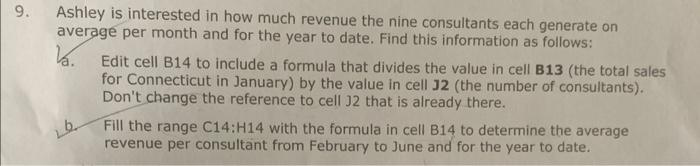Home / Expert Answers / Computer Science / ashley-is-interested-in-how-much-revenue-the-nine-consultants-each-generate-on-average-per-month-an-pa229

# (Solved): Ashley is interested in how much revenue the nine consultants each generate on average per month an ...Ashley is interested in how much revenue the nine consultants each generate on average per month and for the year to date. Find this information as follows: a. Edit cell B14 to include a formula that divides the value in cell B13 (the total sales for Connecticut in January) by the value in cell $$\mathbf{3 2}$$ (the number of consultants). Don't change the reference to cell $$\mathrm{J} 2$$ that is already there. b. Fill the range $$\mathrm{C} 14: \mathrm{H} 14$$ with the formula in cell $$\mathrm{B} 14$$ to determine the average revenue per consultant from February to June and for the year to date.

We have an Answer from Expert

ANSWER: Formula for cell B14 =(B13/$J$2)